• I'm having trouble getting the phase of a simple sine curve using the scipy fft module in python. I followed this tutorial closely and converted the matlab code to python. However, no matter what phas...I'm having trouble getting the phase of a simple sine curve using the scipy fft module in python. I followed this tutorial closely and converted the matlab code to python. However, no matter what phase I use for the input, the graph always shows 3. What am I missing?import numpy as npimport matplotlib.pyplot as pltimport scipy.fftpackimport cmathA=10fc = 10phase=60fs=32#Sampling frequency with oversampling factor of 32t = np.arange(0,2,1/fs)#Convert the phase shift to radians from degrees.phi = phase*np.pi/180x=A*np.cos(2*np.pi*fc*t+phi)N=256X = scipy.fftpack.fftshift(scipy.fftpack.fft(x,N))/Ndf=fs/N #Frequency resolution.sampleindex = np.arange(-N/2,N/2,1) #Ordered index for FFT plot.f = sampleindex*df #x-axis index continued to ordered frequenciesraw_phases = np.angle(X)X2=np.copy(X)#Store the FFT results in another array.#Detect very small numbers and ignore them.tau = max(abs(X))/10X2[abs(X)phase=[cmath.phase(i) for i in X2]plt.plot(f,phase)plt.show()EDIT: Here is some simpler code. Still can't seem to get the phase.y = 28*np.sin(2*np.pi/7*x)yf = scipy.fftpack.fft(y)xf = np.linspace(0.0, 1.0/(2.0*T), N/2)phase = np.angle(yf)yf = np.abs(yf[:N//2])phase = phase[:N//2]xf = xf[1:]yf = yf[1:]phase = phase[1:]yf = yf-np.mean(yf)#The frequencies seem to always be scaled by 0.1433, not sure why.c = 2*np.pi/7/0.143301freqs = xf[yf>np.std(yf)]*cphases = phase[yf>np.std(yf)]The frequencies I get are clustered around 2*np.pi/7. But the phases I get are:array([-0.217795  , -0.22007488, -0.22226087,  2.91723935,  2.91524011,2.91333367])While there should be no phase at all.解决方案This is the simplest code to show how to get the angles.Note that I've created the signal y such that there is an integer number of periods in it (as I suggested in a comment, and @hotpaw2 suggested in their answer).This is the problem with OP's code.I used linspace to create the time axis, using the endpoint=False option. This is important, if t were to contain the number 10, then we no longer have an exact integer number of periods. When working with the discrete Fourier transform, it helps to think of what happens when you repeat the signal. Simply take y, and concatenate a copy of itself: np.hstack((y,y)). Is this new signal still a sampling of a single sine wave, or did we create something more complex? What happens at that point where the two copies meet?import numpy as npimport matplotlib.pyplot as pltimport scipy.fftpackphase = np.pi / 4t = np.linspace(0, 10, num=200, endpoint=False)y = np.cos(2 * np.pi * t + phase)Y = scipy.fftpack.fftshift(scipy.fftpack.fft(y))f = scipy.fftpack.fftshift(scipy.fftpack.fftfreq(len(t)))p = np.angle(Y)p[np.abs(Y) < 1] = 0plt.plot(f, p)plt.show()展开全文• function p = angle(h) %ANGLE Phase angle. % ANGLE(H) returns the phase angles, in radians, of a ... 单位rad，h可以为复数矩阵。 好吧，这个函数其实很简单，但是还是可以学习一下。毕竟自己写也多几个字母呢。
function p = angle(h)
%ANGLE  Phase angle.
%   ANGLE(H) returns the phase angles, in radians, of a matrix with
%   complex elements.
%
%   Class support for input X:
%      float: double, single
%

%   Copyright 1984-2010 The MathWorks, Inc.

p = atan2(imag(h), real(h));

好吧，这个函数其实很简单，但是还是可以学习一下。毕竟自己写也多几个字母呢。

展开全文matlab
• ## 复数与相位(旋转)

千次阅读 2019-09-27 11:39:50
• 此处An和相位角的求法如下： 而对于指数形式的傅里叶变换结果该如何呢？ 固然，直接用傅里叶变换积分来求是一种办法： 但是若此时已经求出三角形式的变换结果了，要转化为指数形式该如何做呢？ 可以根据欧拉公式...
信号表示为三角傅里叶级数时：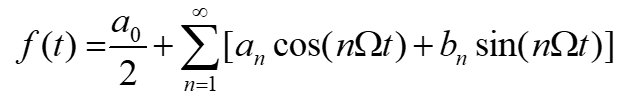以上为进行三角分解后的初步结果，要进行信号的频谱分析还需要将括号内的三角函数合并为余弦的形式。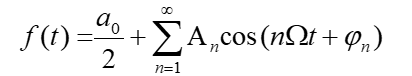此处An和相位角的求法如下：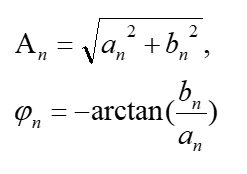而对于指数形式的傅里叶变换结果该如何呢？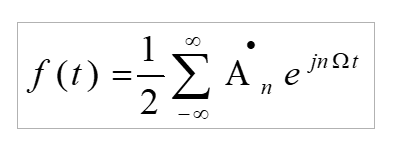固然，直接用傅里叶变换积分来求是一种办法：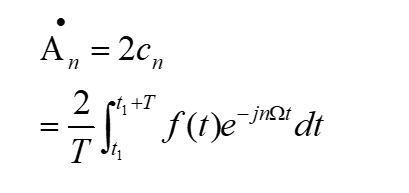但是若此时已经求出三角形式的变换结果了，要转化为指数形式该如何做呢？

可以根据欧拉公式分解：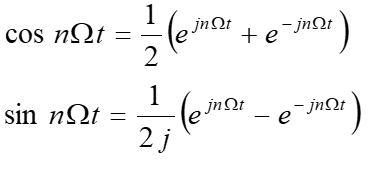例如：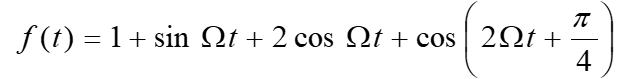此式要转化成指数形式，则：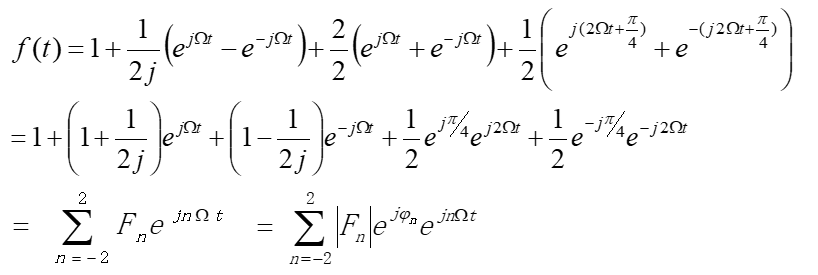问题来了，你怎么知道那个ψn是咋求的啊？这时就不能按照那个前面三角变换中的公式求了，要和复变函数的解法统一起来（用到的知识是求解复数辐角主值的方法）：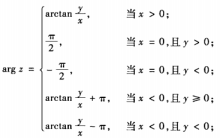展开全文• ## angle--复数的相角

千次阅读 2015-12-28 09:08:49
【功能简介】求复数的相。 【语法格式】 Y=angle(Z)  返回复数的虚部或复数数组中每一个元素的相，单位为弧度，取值范围为[-pi,pi]。对于复数Z，可通过下式计算幅值和相： R=abs(Z); Theta=angle(Z);...
• ## 复数

千次阅读 2019-04-30 14:14:44
复数域是实数域的代数闭包，也即任何复系数多项式在复数域中总有根。 复数是由意大利米兰学者卡当在十六世纪首次引入，经过达朗贝尔、棣莫弗、欧拉、高斯等人的工作，此概念逐渐为数学家所接受。 历史 最早有关复....
• ## 笔记—复数

千次阅读 2020-11-04 16:40:43
在讨论音频频域，即stft变换后的复数时，分别称为幅值和相位。 根据欧拉公式（其中I是虚数符号），可得，这个公式可以方便地把幅值和相位还原回复数，进而做istft，将频域信息转回到时域，即wave波形。 ...机器学习 数学
• 复数是C#中没有的，不能直接调用的。...不过这里包含了复数的基本晕算了了，包括加减乘除、取模运算、计算相位角等！详细信息其直接阅读代码。都包含注释了。 /// <summary> /// 复数类 /// </summar...
• 简单分离实部虚部，或者考虑幅度和相位角都丢失了复数原本的关系。论文按照复数计算的定义，设计了深度复数网络，能对复数的输入数据进行卷积、激活、批规范化等操作。在音频信号的处理中，该网络应该有极大的优势。...深度学习 论文复现 pytorch
• C#复数类Complex的封装 ----------------------------------------------------------------------------------------------------------------------------------------------------------本文作者：随煜而...c# Complex
• 1. 前言 在.net 中没有提供复数类的操作。第三方库Math.net中包含了复数的相关...复数类编写不具有很大难度，但多数复数类均只支持使用实部虚部模式构造一个复数，而幅值相位模式也是构造复数的一个方式。现给出可...c# 算法
• 数组两种表示方法 ...复数数组 for m=1:2 for n=1:2 Am1(m,n)=abs(A(m,n)); Aa1(m,n)=angle(A(m,n))*180/pi; end end Am1,Aa1 >> test Am1 = 5.0990 7...
• 复数的表示 复数：首先，复数基本单位是 i=−1i=\sqrt{−1}i=−1​。 有了这个单位，复数空间中的每个数都可以表示为 a+bia+bia+bi 的形式。其中，a 被称为实部 real part，b 被称为虚部 imaginary part。 复数...
• ## 复数与相量法

千次阅读 多人点赞 2018-09-05 16:49:13
1. 复数的几种表示形式 实部、虚部（直角坐标系）： （a是实部，b是虚部） 幅值、相（指数形式）： （r是幅值，θ 是相 ） 两种形式相互转换： 【证明】 若 ，则 所以 ，实部 ，虚部 幅值 ，相 ...
• 对于一般对线条件，此代码“qr_lapack”支持在 QR 分解中允许任何给定的对角复数相位，例如所有非负值，与原始矩阵 A 中的相同，或任何其他。 例如， DIAG_VEC = 诊断（A）； [Q, R] = qr_lapack (A, ECON, DIAG_...matlab
• 2、real - 复数的实部 此 MATLAB 函数 返回复数数组 Z 元素的实部。 3、imag - 复数的虚部 此 MATLAB 函数 返回数组 Z 的元素的虚部。 4、abs - 绝对值和复数幅值 此 MATLAB 函数 返回数组 X 中每个元素...matlab
• 对于复数，angle()是求相位角，取值范围是-pi到pi； abs()对于实数是求绝对值，对于复数是求其模值，X为一复数，abs(X)=sqrt(real(X).^2 + imag(X).^2)； 另有：Z为一个复数时，R=abs(Z)，theta=angle(Z),之后利用...matlab angle函数 abs函数
• 文章目录复数复数常规算术运算复数操作函数分数分数常规算术运算分数算术函数分数转换成浮点数 julia 不仅支持数学运算以及基本函数 ，还支持复数和分数相关的算术操作。 复数 在Julia中, im 用来表示复数 i，表示 -...
• MATLAB笔记之复数基本公式 QQ:3020889729 小蔡复数的创建复数的实部与虚部的获取复数的辐角与模的获取复数的共轭运算 QQ:30...matlab
• 2、用法说明：p=angle(z)函数计算复数z的相位角p。  输出的结果p与输入的z的维数相同，返回值为复数数组z中的每个元素的相位角，单位为弧度。  其值均在正负pi之间 3、实例 >> x = [2-4i 7-9i 23+12i 98-...Matlab angle函数
• 创建一个复数类complex，私有成员包括实部real和虚部imag，初始化使用 init成员函数，计算幅值使用amplitude成员函数，计算相使用phase成员函数， 同时为complex定义加减乘除四则运算函数。 /*创建一个复数类...c++基础
• 最近因为项目需要，需要写一段复数计算的程序，项目是用C语言写的，最初想的是自己定义一个复数类型，包含实部虚部，但是完成基本运算就麻烦些，不想使用C++的话可以直接进行操作符重载，这样计算的算法是可以通用的...c语言 complex
• MATLAB程序 a=3+4*i %复数 real(a) %求复数的实部 ...angle(a) %求复数相位 conj(a) %求复数的复共轭 　 %% Irradiance and phase resulting figure subplot(2,2,1) mesh(2.*xn./D2,2.*yn...
• 复数的本质是一种旋转，不要只记住i=−1i=\sqrt{-1}i=−1​,在复平面中，从1到i就是逆时针旋转90度，即乘i等于逆时针，乘-1等于顺时针，两次-i就正好等于两次i。有了这一层思想后，对复数。能有更深的认识 eπi+1=0e...线性代数 抽象代数
• 这个模块提供了对复数数学函数的访问。此模块中的函数接受整数、浮点数或复数作为参数。它们还将接受任何具有_complex__()或_float__()方法的Python对象:这些方法分别用于将对象转换为复数的或浮点数，然后将该函数...
• 简而言之，与希尔伯特函数时域卷积，是在频域将频谱搬移pi/2的弧度，相位移动90°，而后者频域的变化才是我们研究的重点。即经过希尔伯特变换之后正弦函数->余弦函数，余弦函数->正弦函数 那么我们由下图可知...
• end https://www.zhihu.com/video/1248921905019019264 5.求复数的模和相位 w=linspace(-10,10,5000); y=power(i*w,2); f=power(y+1,-1); u=abs(f); u1=angle(f); plot(w,u); >> plot(w,u1); 6.拉氏变换三维图 二维......